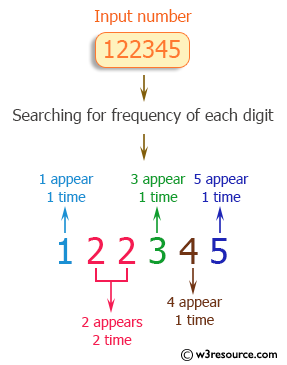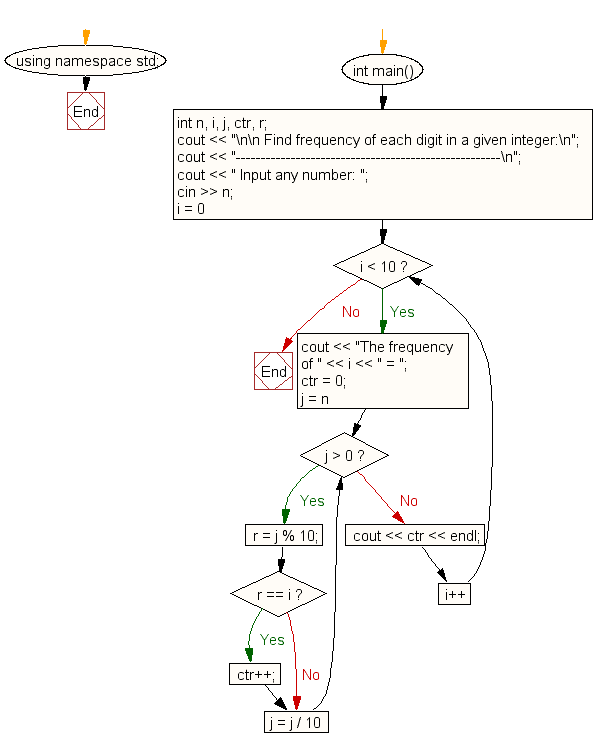﻿ C++ : Find the frequency of each digit in a given integer# C++ Exercises: Find the frequency of each digit in a given integer

## C++ For Loop: Exercise-59 with Solution

Write a program in C++ to find the frequency of each digit in a given integer.

Pictorial Presentation:Sample Solution:-

C++ Code :

``````#include <iostream>
using namespace std;
int main()
{
int n, i, j, ctr, r;
cout << "\n\n Find frequency of each digit in a given integer:\n";
cout << "-----------------------------------------------------\n";
cout << " Input any number: ";
cin >> n;
for (i = 0; i < 10; i++)
{
cout << "The frequency of " << i << " = ";
ctr = 0;
for (j = n; j > 0; j = j / 10)
{
r = j % 10;
if (r == i)
{
ctr++;
}
}
cout << ctr << endl;
}
}
``````

Sample Output:

``` Find frequency of each digit in a given integer:
-----------------------------------------------------
Input any number: 122345
The frequency of 0 = 0
The frequency of 1 = 1
The frequency of 2 = 2
The frequency of 3 = 1
The frequency of 4 = 1
The frequency of 5 = 1
The frequency of 6 = 0
The frequency of 7 = 0
The frequency of 8 = 0
The frequency of 9 = 0
```

Flowchart:C++ Code Editor:

Contribute your code and comments through Disqus.

What is the difficulty level of this exercise?

﻿